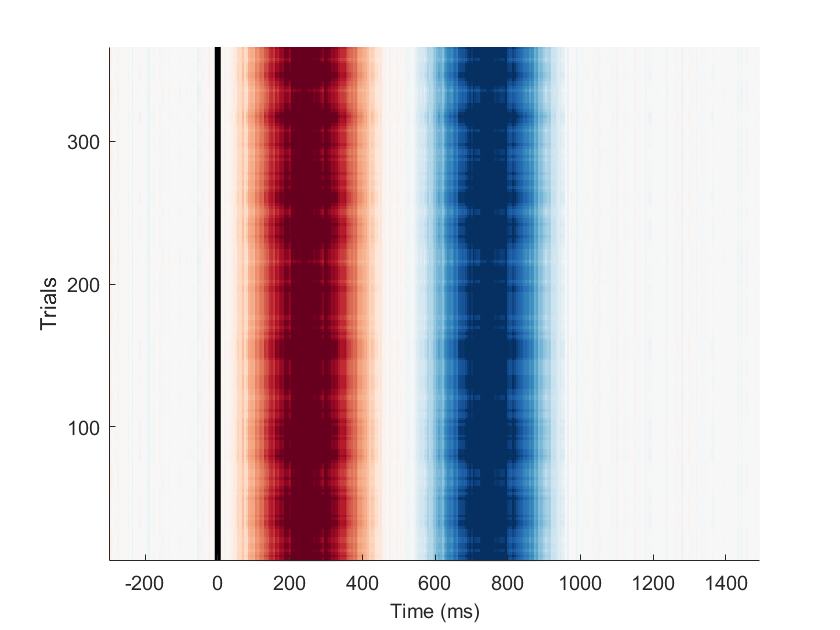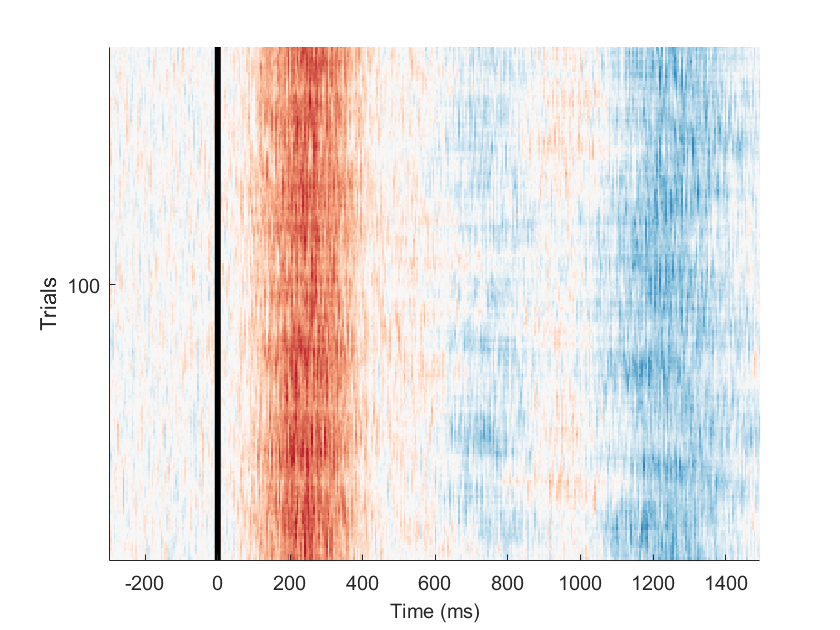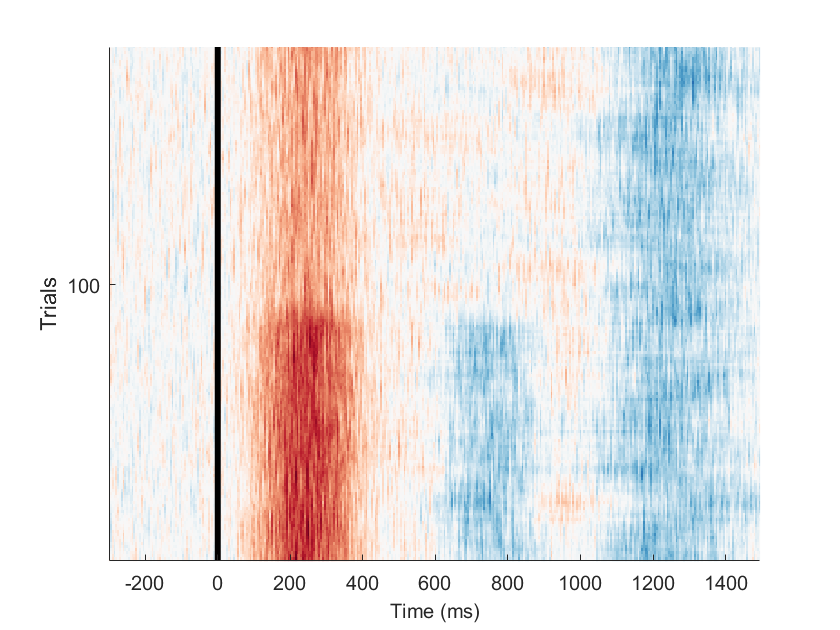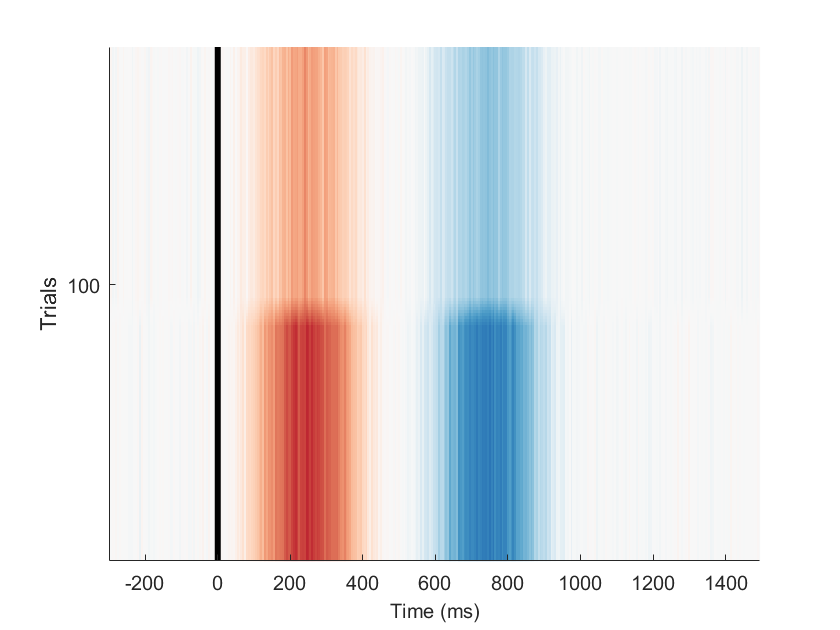init_unfold
Starting unfold toolbox. Adding subfolders and other toolboxes to path... Done.

# Plotting Single-Trial ERPimages

% We simulate data with a stimulus onset of two types + a buttonpress
EEG = tutorial_simulate_data('erpimage');
eeg_checkset note: upper time limit (xmax) adjusted so (xmax-xmin)*srate+1 = number of frames Event resorted by increasing latencies.
This design has Stimulus and Buttonpress. The stimulus has two levels: face and house. There is only overlap between stimulus and buttonpress, but not between buttonpress and subsequent stimulus..
cfgDesign = [];
cfgDesign.eventtypes = {'stimulus','buttonpress'};
cfgDesign.formula = {'y ~ 1+ cat(stimulusType)','y~1'};
EEG = uf_designmat(EEG,cfgDesign);
Multiple events with separate model-formula detected Modeling 185 event(s) of [stimulus] using formula: y~1+stimulusType Modeling 185 event(s) of [buttonpress] using formula: y~1
cfgTimeexpand = [];
cfgTimeexpand.timelimits = [-.3,1.5];
EEG = uf_timeexpandDesignmat(EEG,cfgTimeexpand);
uf_timeexpandDesignmat(): Timeexpanding the designmatrix... ...done
EEG= uf_glmfit(EEG);
uf_glmfit(): Fitting deconvolution model...solving the equation system solving electrode 1 (of 1 electrodes in total)... 30 iterations, took 0.0s LMfit finished
After fitting the EEG, we can visualize the ERPimages
uf_erpimage(EEG,'channel',1)
pop_epoch():370 epochs selected Epoching... pop_epoch():370 epochs generated eeg_checkset note: event field format 'stimulusType' made uniform pop_epoch(): checking epochs for data discontinuity Recalculating the EEG_epoch field using eeg_checkset, might take some time Aligning erpimage to event , 370 epochs found Sorting erpimage from event forwards by field latency Plotting input data as 370 epochs of 360 frames sampled at 200.0 Hz. Sorting data on input sortvar. 100.00% of the trials (i.e., 370 out of 370) have the same sortvar value as at least one other trial. Distribution of number ties per unique value of sortvar: Min: 370, 25th ptile: 370, Median: 370, 75th ptile: 370, Max: 370 Smoothing the sorted epochs with a 10-epoch moving window. and a decimation factor of 1 Using the specified caxis range of [-2.35367,2.35367]. Data will be plotted between -300 and 1495 ms.Output data will be 360 frames by 361 smoothed trials. Outtrials: 6.00 to 366.00 Overplotting sorted sortvar on data. Done.
By default the erpimage is not sorted and the plot is depicting the overlap-corrected estimate.

### CFG-Trick

to increase legibility, I am going to introduce some plotting options to each plot by constructing a cell-array suitable to pass to each of the following erpimages
cfg = []
cfg = []
cfg.channel = 1
cfg = struct with fields:
channel: 1
cfg.figure = 1
cfg = struct with fields:
channel: 1 figure: 1
cfg.caxis = [-5,5]
cfg = struct with fields:
channel: 1 figure: 1 caxis: [-5 5]
cfg.alignto = 'stimulus'
cfg = struct with fields:
channel: 1 figure: 1 caxis: [-5 5] alignto: 'stimulus'
cfg = [fieldnames(cfg),struct2cell(cfg)].';
cfg(:)
ans = 8×1 cell array
{'channel' } {[ 1]} {'figure' } {[ 1]} {'caxis' } {1×2 double} {'alignto' } {'stimulus'}

## Raw ERPimage

We can also look at the raw ERPimage. This is the same as running eeg_erpimage on your typical epoched data. As you can see, compared to the overlap-corrected model-estimate, we have much more noise included here.
uf_erpimage(EEG,'type','raw',cfg{:})
pop_epoch():370 epochs selected Epoching... pop_epoch():370 epochs generated eeg_checkset note: event field format 'stimulusType' made uniform pop_epoch(): checking epochs for data discontinuity Recalculating the EEG_epoch field using eeg_checkset, might take some time Aligning erpimage to event stimulus, 185 epochs found Sorting erpimage from event stimulus forwards by field latency Plotting input data as 185 epochs of 360 frames sampled at 200.0 Hz. Sorting data on input sortvar. 100.00% of the trials (i.e., 185 out of 185) have the same sortvar value as at least one other trial. Distribution of number ties per unique value of sortvar: Min: 185, 25th ptile: 185, Median: 185, 75th ptile: 185, Max: 185 Smoothing the sorted epochs with a 10-epoch moving window. and a decimation factor of 1 Using the specified caxis range of [-5,5]. Data will be plotted between -300 and 1495 ms.Output data will be 360 frames by 176 smoothed trials. Outtrials: 6.00 to 181.00 Overplotting sorted sortvar on data. Done.

## Sorting trials by condition

Now we can start sorting trials by condition, for instance here by stimulus type.
uf_erpimage(EEG,'type','raw','sort_by','stimulusType',cfg{:})
pop_epoch():370 epochs selected Epoching... pop_epoch():370 epochs generated eeg_checkset note: event field format 'stimulusType' made uniform pop_epoch(): checking epochs for data discontinuity Recalculating the EEG_epoch field using eeg_checkset, might take some time Aligning erpimage to event stimulus, 185 epochs found Sorting erpimage from event stimulus forwards by field stimulusType Plotting input data as 185 epochs of 360 frames sampled at 200.0 Hz. Sorting data on input sortvar. 100.00% of the trials (i.e., 185 out of 185) have the same sortvar value as at least one other trial. Distribution of number ties per unique value of sortvar: Min: 90, 25th ptile: 90, Median: 93, 75th ptile: 95, Max: 95 Smoothing the sorted epochs with a 10-epoch moving window. and a decimation factor of 1 Using the specified caxis range of [-5,5]. Data will be plotted between -300 and 1495 ms.Output data will be 360 frames by 176 smoothed trials. Outtrials: 6.00 to 181.00 Overplotting sorted sortvar on data. Done.
We directly see, that one condition seems to have a smaller effect than the other (based on the "P2" at 200ms. But towards the end of the epoch there is another interesting pattern. Let's investigate this further. We switch back the type to modelled in order to see what the overlap contributes to this pattern.
uf_erpimage(EEG,'type','modelled','sort_by','stimulusType',cfg{:})
pop_epoch():370 epochs selected Epoching... pop_epoch():370 epochs generated eeg_checkset note: event field format 'stimulusType' made uniform pop_epoch(): checking epochs for data discontinuity Recalculating the EEG_epoch field using eeg_checkset, might take some time Aligning erpimage to event stimulus, 185 epochs found Sorting erpimage from event stimulus forwards by field stimulusType Plotting input data as 185 epochs of 360 frames sampled at 200.0 Hz. Sorting data on input sortvar. 100.00% of the trials (i.e., 185 out of 185) have the same sortvar value as at least one other trial. Distribution of number ties per unique value of sortvar: Min: 90, 25th ptile: 90, Median: 93, 75th ptile: 95, Max: 95 Smoothing the sorted epochs with a 10-epoch moving window. and a decimation factor of 1 Using the specified caxis range of [-5,5]. Data will be plotted between -300 and 1495 ms.Output data will be 360 frames by 176 smoothed trials. Outtrials: 6.00 to 181.00 Overplotting sorted sortvar on data. Done.
Interesting, only the first two peaks seem to be associated with the stimulus. Where do the later peaks come from?

## Sorting trials by event

Let's sort by the next trial onset. For this we have to specify sort_time to sort by something larger than 0.
uf_erpimage(EEG,'type','raw','sort_time',[eps 1.5],'sort_alignto',{'buttonpress'},cfg{:})
pop_epoch():370 epochs selected Epoching... pop_epoch():370 epochs generated eeg_checkset note: event field format 'stimulusType' made uniform pop_epoch(): checking epochs for data discontinuity Recalculating the EEG_epoch field using eeg_checkset, might take some time Aligning erpimage to event stimulus, 185 epochs found Sorting erpimage from event buttonpress forwards by field latency Plotting input data as 185 epochs of 360 frames sampled at 200.0 Hz. Sorting data on input sortvar. 85.41% of the trials (i.e., 158 out of 185) have the same sortvar value as at least one other trial. Distribution of number ties per unique value of sortvar: Min: 0, 25th ptile: 0, Median: 2, 75th ptile: 4, Max: 7 Smoothing the sorted epochs with a 10-epoch moving window. and a decimation factor of 1 Using the specified caxis range of [-5,5]. Data will be plotted between -300 and 1495 ms.